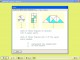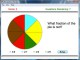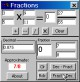Fraction Shape-Up 1.00 Interactive software that helps students understand and work with fractions. SharewareFraction Fusion 2.1 Fraction Fusion teaches and reviews fraction concepts to middle elementary-aged students. DemoFractionAction 0.9 FractionAction will do the following: reduce fractions; fraction arithmetic; decimal to/from fraction conversions with periodic (repeating) digits displayed; fraction to/from continued fraction conversions for common fractions and fractions with simple square roots. SharewareFraction Fonts 2.0 Fraction Fonts are a handy way for Mac-based Desktop Publishers to add the most commonly used fractions to their documents. DemoAbilities Builder Fraction Facts 3.5 Build fraction reducing and simplifying skills for simple to complex problems. DemoFraction Squares 1.0 Fraction Squares shows a series of 160 pre-set drawings. FreewareFRS Fraction Master 2.1 Learn fractions faster. SharewareFractions n Decimals CE 5.3 Add, Subtract, Divide and Multiply fractions. Convert decimals/fractions CE 2.11 SharewareFraction Plugin 1.0 Fraction Plugin is a plugin for REALbasic 5 which, because of operator and function overloading, allows fractions to be used much like doubles and integers in REALbasic programs. FreewareAbilities Builder Add & Subtract Fractions 3.1 Build fraction addition and subtraction skills for simple to complex problems. Demo